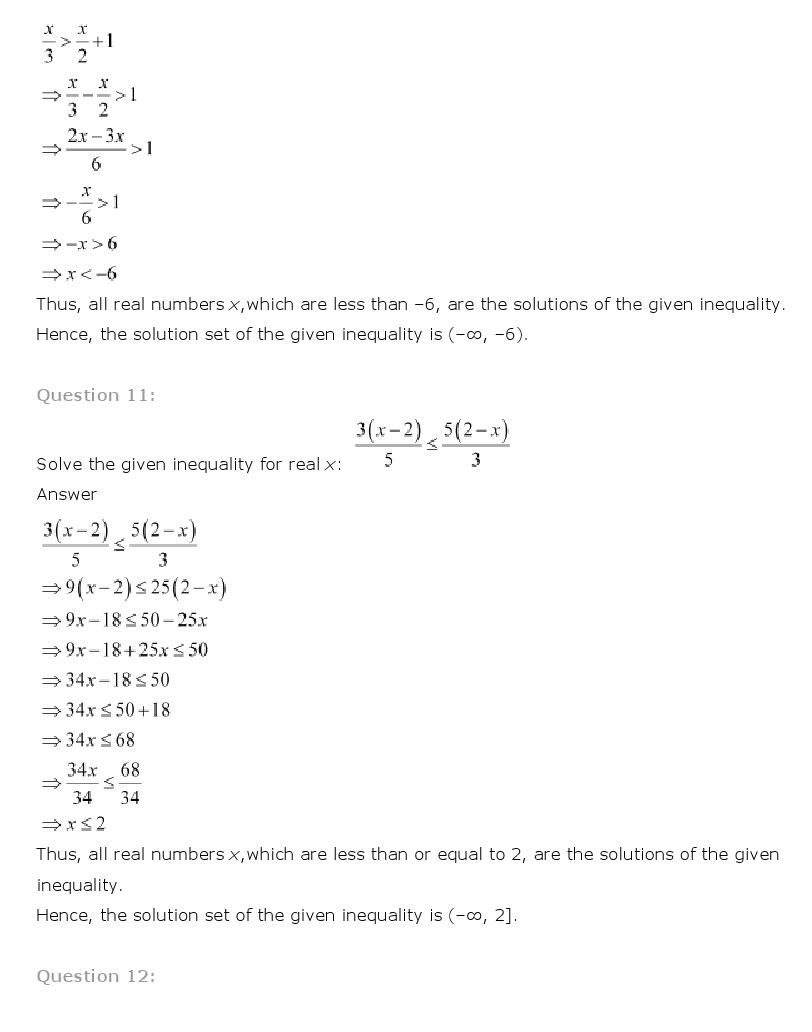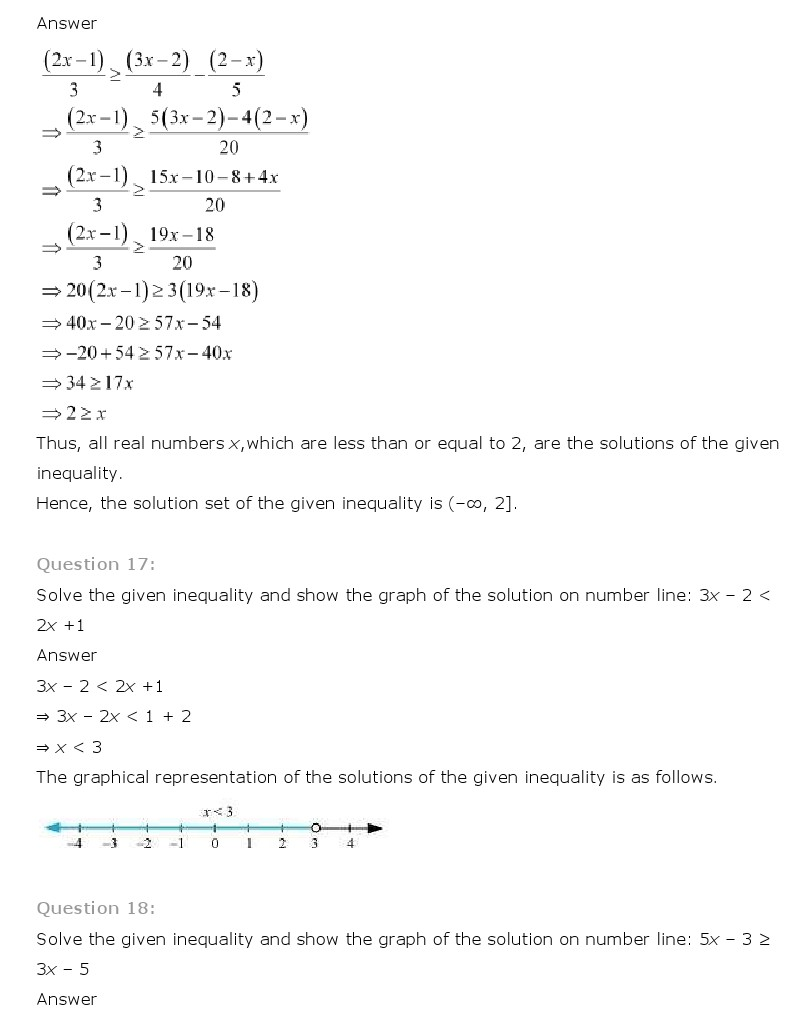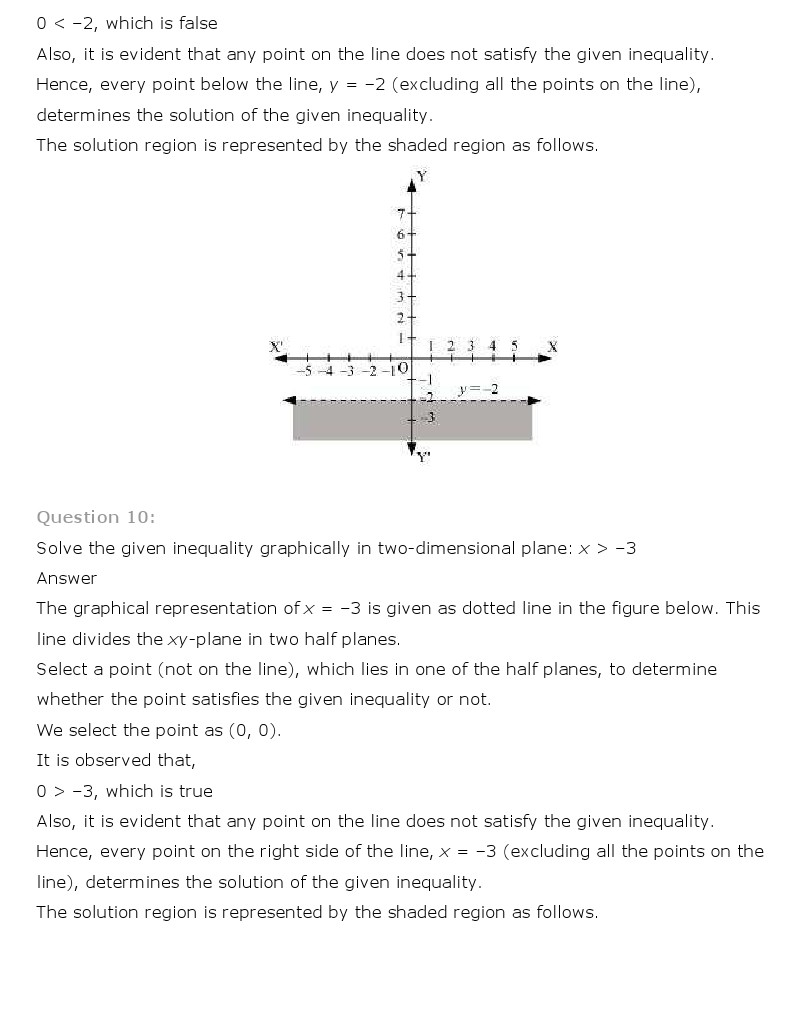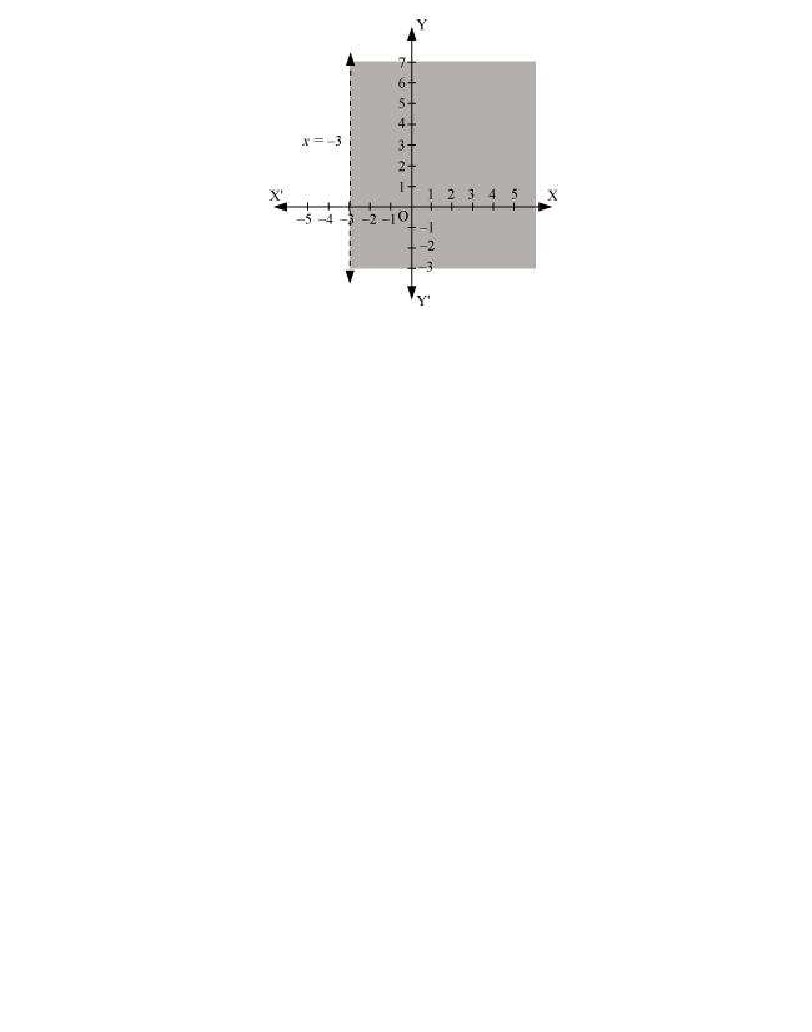# NCERT Solutions for Class 11th Maths Chapter 6 Linear Inequalities

NCERT Solutions for Class 11th Maths Chapter 6 Linear Inequalities

## NCERT Solutions for Class 11th Maths Chapter 6 Linear Inequalities

National Council of Educational Research and Training (NCERT) Book Solutions for class 11th
Subject: Maths
Chapter: Chapter 6 Linear Inequalities

Class 11th Maths Chapter 6 Linear Inequalities NCERT Solution is given below.CBSE sample papers http://www.cbsesamplepapers.info/
RD sharma solutions for class 11: http://www.cbselabs.com/forums/rd-sharma-class-11-solutions.46/

NCERT Solutions for maths in Video https://www.youtube.com/user/cbsepapers/videos
Solving linear inequalities is almost exactly like solving linear equations.

Solve x + 3 < 0. If they'd given me "x + 3 = 0", I'd have known how to solve: I would have subtracted 3 from both sides. I can do the same thing here: x < -3 Then the solution is: x < –3 "Interval notation" writes the solution as an interval (that is, as a section or length along the number line). The above solution, "x < –3", would be written as "(-infinity, -3)", which is pronounced as "the interval from negative infinity to minus three", or just "minus infinity to minus three". Interval notation is easier to write than to pronounce, because of the ambiguity regarding whether or not the endpoints are included in the interval. (To denote, for instance, "x < –3", the interval would be written "(-infinity, -3]", which would be pronounced as "minus infinity through (not just "to") minus three" or "minus infinity to minus three, inclusive", meaning that –3 would be included. The right-parenthesis in the "x < –3" case indicated that the –3 was not included; the right-bracket in the "x < –3" case indicates that it is.) The last "notation" is more of an illustration. You may be directed to "graph" the solution. This means that you would draw the number line, and then highlight the portion that is included in the solution. First, you would mark off the edge of the solution interval, in this case being –3. Since –3 is not included in the solution (this is a "less than", remember, not a "less than or equal to"), you would mark this point with an open dot or with an open parenthesis pointing in the direction of the rest of the solution interva